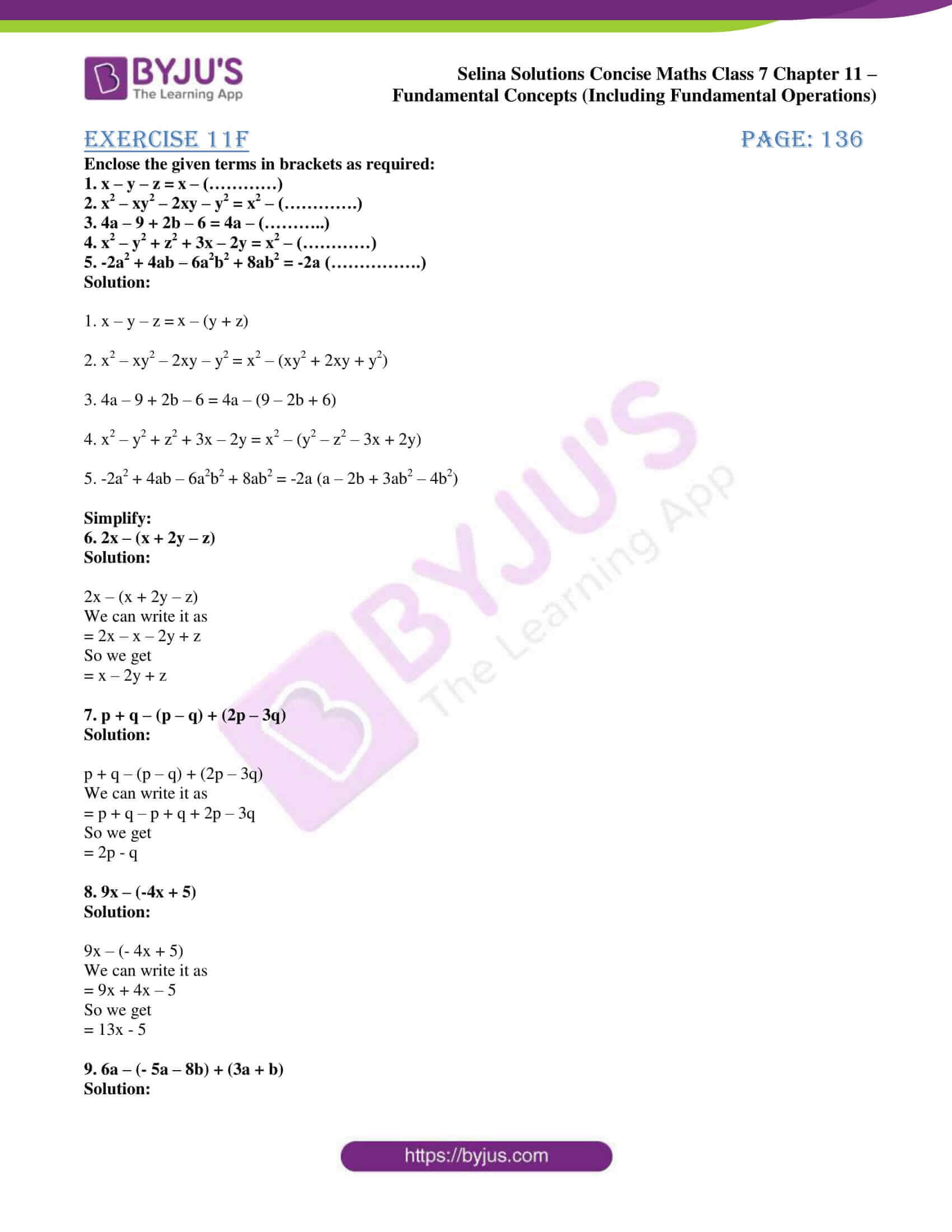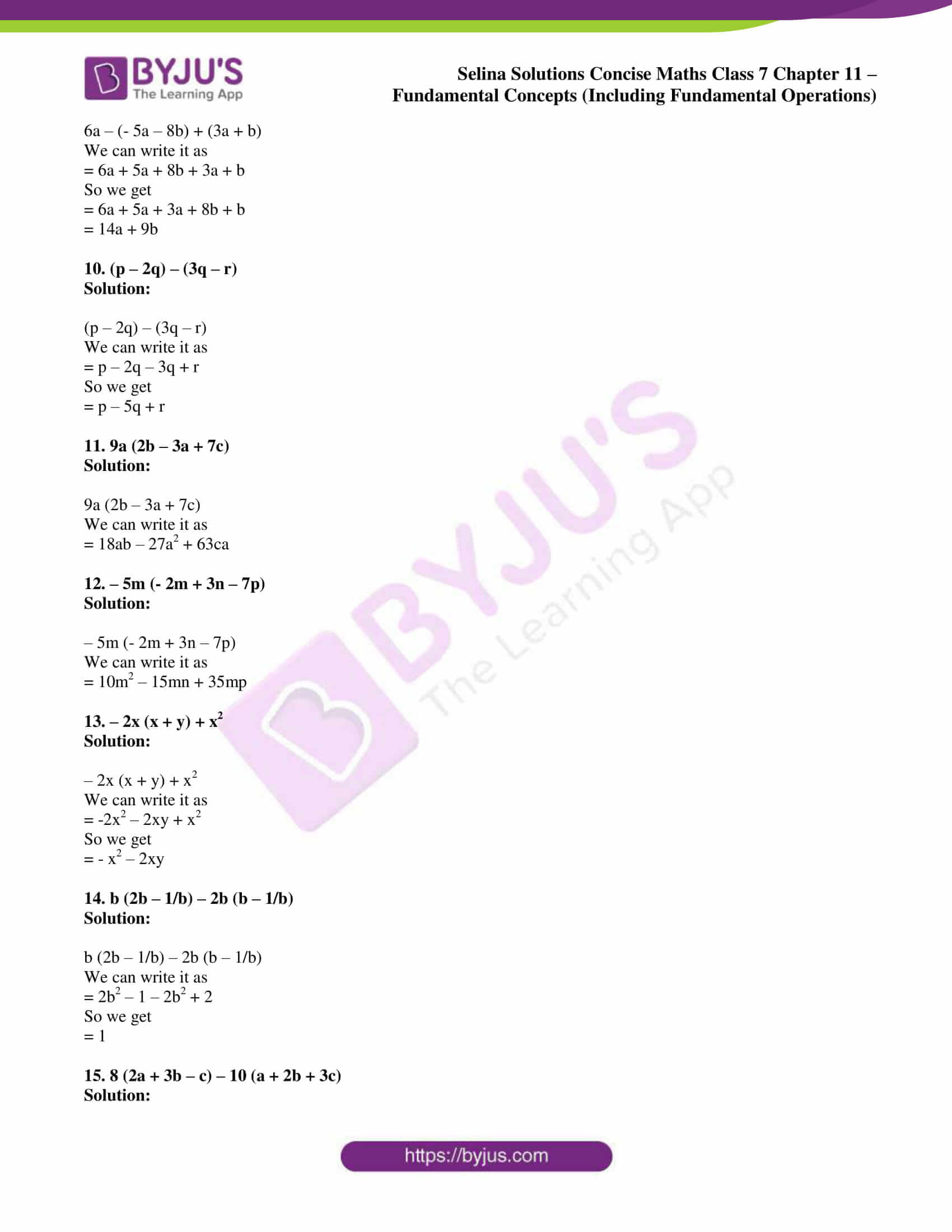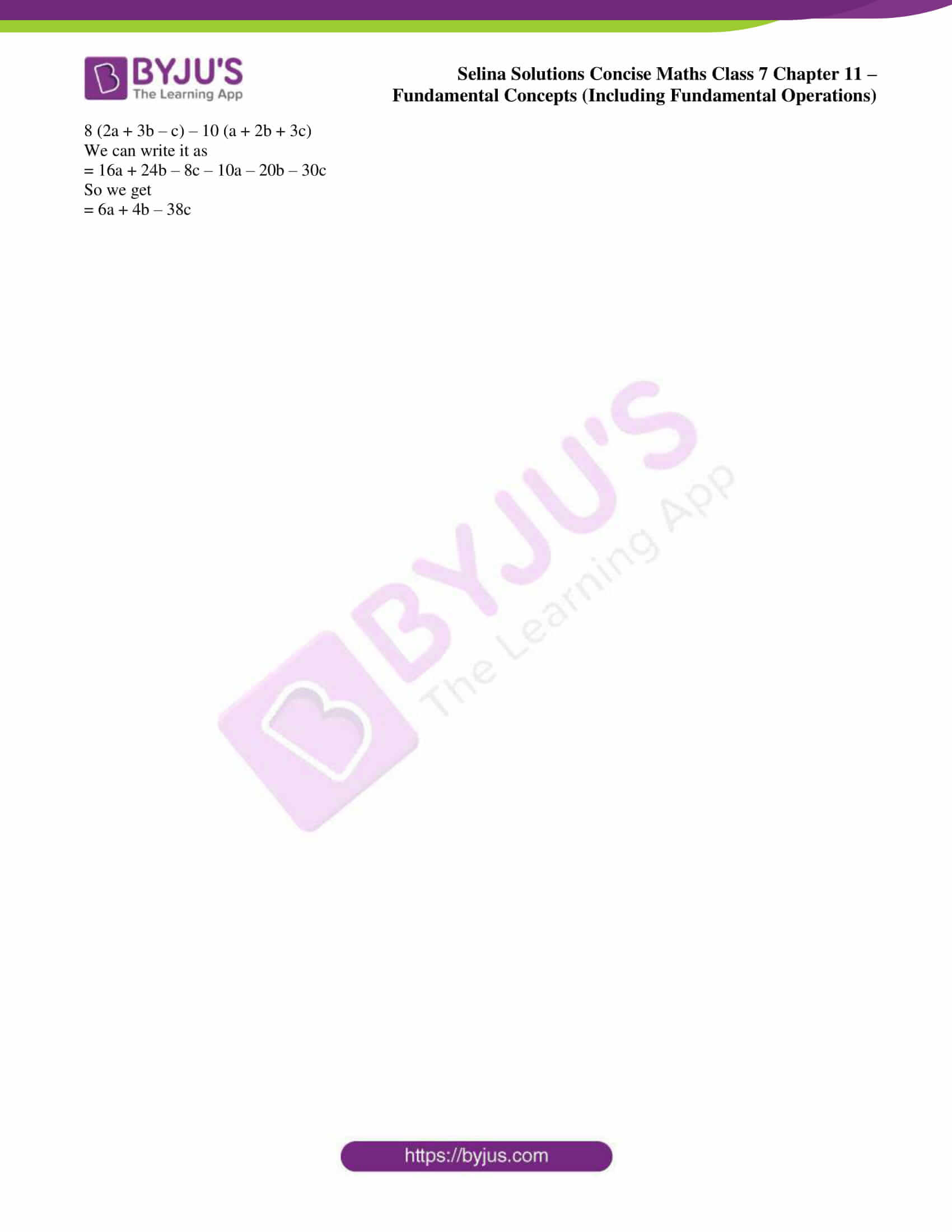# Selina Solutions Concise Maths Class 7 Chapter 11: Fundamental Concepts (Including Fundamental Operations) Exercise 11F

Selina Solutions Concise Maths Class 7 Chapter 11 Fundamental Concepts (Including Fundamental Operations) Exercise 11F are designed by subject matter experts at BYJU’S, by keeping in mind the grasping abilities of students. Using brackets, removal of brackets and types of brackets are the major concepts, which are explained in brief under this exercise. Students who aspire to score well in the Class 7 annual exam are advised to solve the textbook questions referring to the solutions PDF. Selina Solutions Concise Maths Class 7 Chapter 11 Fundamental Concepts (Including Fundamental Operations) Exercise 11F, PDF can be downloaded from the links which are available below.

## Selina Solutions Concise Maths Class 7 Chapter 11: Fundamental Concepts (Including Fundamental Operations) Exercise 11F Download PDF### Access other exercises of Selina Solutions Concise Maths Class 7 Chapter 11: Fundamental Concepts (Including Fundamental Operations)

Exercise 11A Solutions

Exercise 11B Solutions

Exercise 11C Solutions

Exercise 11D Solutions

Exercise 11E Solutions

### Access Selina Solutions Concise Maths Class 7 Chapter 11: Fundamental Concepts (Including Fundamental Operations) Exercise 11F

#### Exercise 11F page: 136

Enclose the given terms in brackets as required:

1. x – y – z = x – (…………)

2. x2 – xy2 – 2xy – y2 = x2 – (………….)

3. 4a – 9 + 2b – 6 = 4a – (………..)

4. x2 – y2 + z2 + 3x – 2y = x2 – (…………)

5. -2a2 + 4ab – 6a2b2 + 8ab2 = -2a (…………….)

Solution:

1. x – y – z = x – (y + z)

2. x2 – xy2 – 2xy – y2 = x2 – (xy2 + 2xy + y2)

3. 4a – 9 + 2b – 6 = 4a – (9 – 2b + 6)

4. x2 – y2 + z2 + 3x – 2y = x2 – (y2 – z2 – 3x + 2y)

5. -2a2 + 4ab – 6a2b2 + 8ab2 = -2a (a – 2b + 3ab2 – 4b2)

Simplify:

6. 2x – (x + 2y – z)

Solution:

2x – (x + 2y – z)

We can write it as

= 2x – x – 2y + z

So we get

= x – 2y + z

7. p + q – (p – q) + (2p – 3q)

Solution:

p + q – (p – q) + (2p – 3q)

We can write it as

= p + q – p + q + 2p – 3q

So we get

= 2p – q

8. 9x – (-4x + 5)

Solution:

9x – (- 4x + 5)

We can write it as

= 9x + 4x – 5

So we get

= 13x – 5

9. 6a – (- 5a – 8b) + (3a + b)

Solution:

6a – (- 5a – 8b) + (3a + b)

We can write it as

= 6a + 5a + 8b + 3a + b

So we get

= 6a + 5a + 3a + 8b + b

= 14a + 9b

10. (p – 2q) – (3q – r)

Solution:

(p – 2q) – (3q – r)

We can write it as

= p – 2q – 3q + r

So we get

= p – 5q + r

11. 9a (2b – 3a + 7c)

Solution:

9a (2b – 3a + 7c)

We can write it as

= 18ab – 27a2 + 63ca

12. – 5m (- 2m + 3n – 7p)

Solution:

– 5m (- 2m + 3n – 7p)

We can write it as

= 10m2 – 15mn + 35mp

13. – 2x (x + y) + x2

Solution:

– 2x (x + y) + x2

We can write it as

= -2x2 – 2xy + x2

So we get

= – x2 – 2xy

14. b (2b – 1/b) – 2b (b – 1/b)

Solution:

b (2b – 1/b) – 2b (b – 1/b)

We can write it as

= 2b2 – 1 – 2b2 + 2

So we get

= 1

15. 8 (2a + 3b – c) – 10 (a + 2b + 3c)

Solution:

8 (2a + 3b – c) – 10 (a + 2b + 3c)

We can write it as

= 16a + 24b – 8c – 10a – 20b – 30c

So we get

= 6a + 4b – 38c